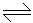# Chemical Engineering - Chemical Reaction Engineering - Discussion

1.

An isothermal aqueous phase reversible reaction, PR, is to be carried out in a mixed flow reactor. The reaction rate in k.mole/m3 .h is given by, r = 0.5CP - 0.125CR. A stream containing only P enters the reactor. The residence time required (in hours) for 40% conversion of P is

 [A]. 0.8 [B]. 1.33 [C]. 1.6 [D]. 2.67

Explanation:

No answer description available for this question.

 Rohith said: (Jun 5, 2019) The correct Answer is B.

 Moazzam said: (May 4, 2020) Please give an explanation of the answer.

 M. Manoj Kumar said: (May 4, 2020) Cp=Cpo(1-x). Cq=Cqo + 1/1Cpo(x). Cqo=0. (T/Cpo ) =x/rp, X=0.4. Solving and eliminating Cpo, we get T=8/5 =1.6.